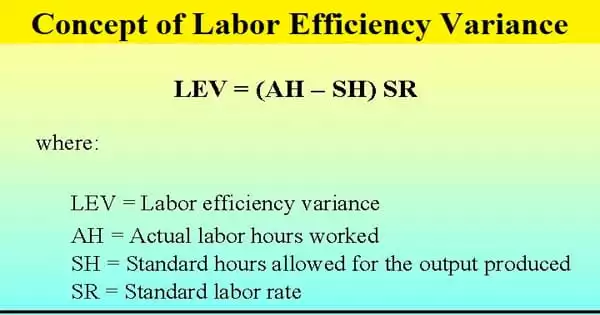Accounting

# Concept of Labor Efficiency VarianceVariance is a statistical measurement term that describes the difference between individual numbers in a data set and the mean of that data set. The labor efficiency variance assesses a company’s ability to use labor in accordance with expectations. It is the difference between the labor hours specified for the activity completed and the actual labor hours expected for a given level of output. The variance is useful for highlighting areas of the manufacturing process that are consuming more labor hours than expected. It is also known as time variance, and it is the result of taking more or less time than the standard time specified for completing the work.

The labor efficiency variance measures the difference between the actual number of hours it takes to complete an activity and the standard number of hours labor should take to complete that activity. The standard number of hours represents a company’s industrial engineers’ best estimate of the optimal speed at which the production staff can manufacture goods. The labor efficiency variance is calculated as follows:

(Actual hours – Standard hours) x Standard rate = Labor efficiency variance

An unfavorable variance means that labor efficiency has worsened, and a favorable variance means that labor efficiency has increased.

Formula for the Calculation of Labor Efficiency Variance (LEV) –

LEV = SR x (ST-AT)

Where,

• LEV = Labor efficiency variance
• SR = Standard rate
• ST = Standard time
• AT = Actual time
• OR, LEV = LMV+LYV+LITV

Where,

• LMV = Labor mix variance
• LYV = Labor yield variance
• LITV = Labor idle time variance

If the actual time is less than the standard time, there will be favorable variance; if the actual time is greater than the standard time, there will be unfavorable variance. To properly understand the labor efficiency variance, you must first understand the concept and operation of standard costing. Variance is simply a method used in the context of standard costing.

Example

During the development of its annual budget, Hodgson Industrial Design’s industrial engineers decide that the standard amount of time required to produce a green widget should be 30 minutes, based on certain assumptions about the efficiency of Hodgson’s production staff, the availability of materials, capacity availability, and so on. During the month, widget materials were in short supply, so Hodgson had to pay production staff even when there was no material to work on, resulting in an average production time per unit of 45 minutes. During the month, the company manufactured 1,000 widgets. Because the standard labor hour cost is \$20, the labor efficiency variance is calculated as follows:

(750 Actual hours – 500 Standard hours) x \$20 Standard rate = \$5,000 Labor efficiency variance.